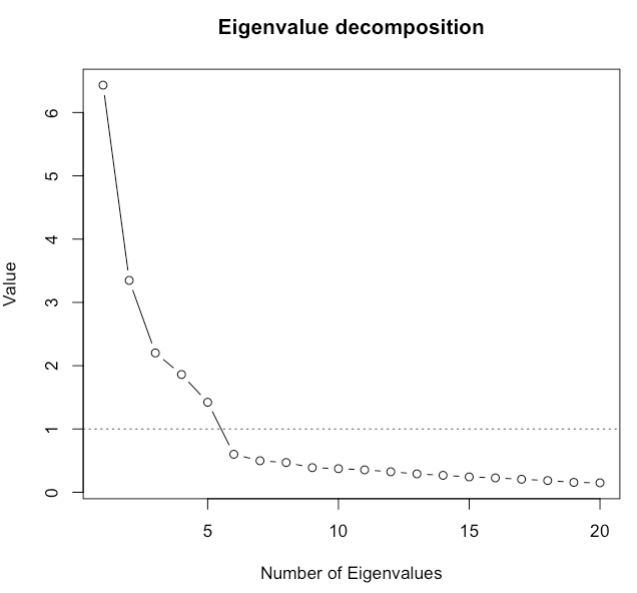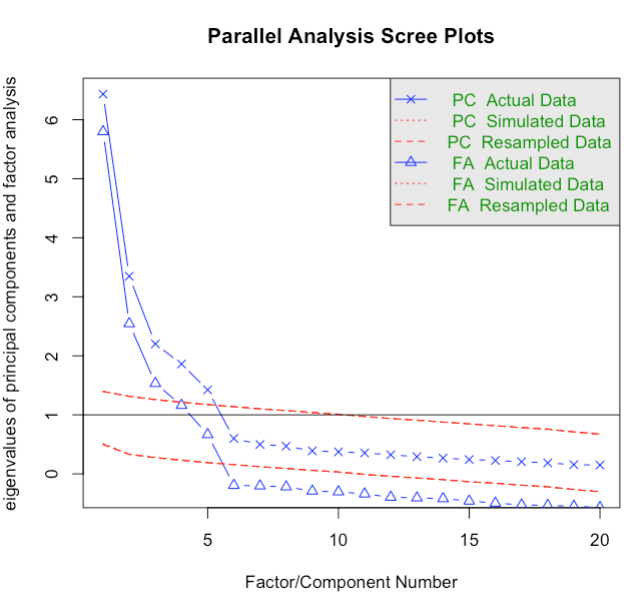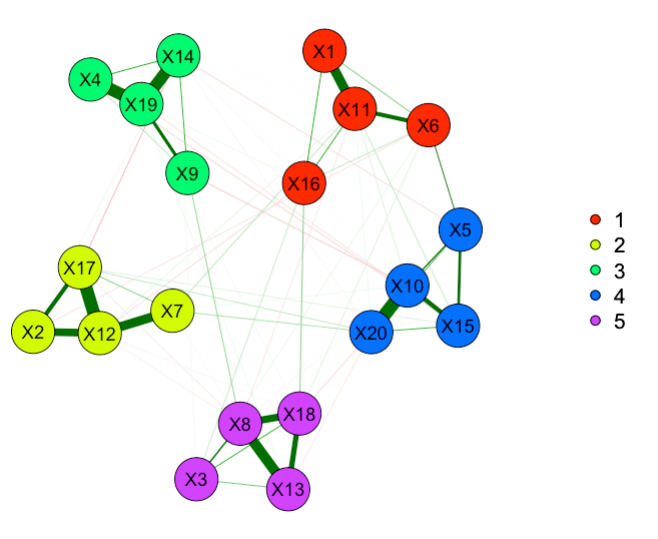# Creating basic psychometric summaries in R

We just finished collecting the first cohort of the WARN-D study, our ambitious effort to create a personalized early warning system for depression. In the 90 minute self report survey, we try to get at many of the most relevant predicators for depression onset. Creating this battery took us many months, and involved a delphi study of experts.

I’m now looking into the data for the first time, and to save myself a bit of time, I created a basic R script to get rough overviews for each scale. This script uses a number of tools (exploratory and confirmatory factor analysis, principal component analysis, network analysis) to get at basic information. I thought I’d share it here if others wanted to use it. It’s set up in a way that all you need to do is select your particular items, and it should all just run automatically.

I’ll walk you through the code briefly, and paste some graphs of how this looks like in example data. In the models, we’re going to ignore distributions (skewed), ordinal vs gaussian data (I just assume data to be normal for this example), and any complications: this is really just a quick setup.

(Note: WordPress keeps screwing with my assign symbol, so I’ll use “←” here instead.)

## Step 1: packages and basic data wrangling

```library("readr") library("dplyr") library("psych") library("qgraph") library("bootnet") library("OpenMx") library("EGAnet") library("lavaan")```

I show you two ways to select subsets of your data. First, when variables are organized subsequently.

```df ← read_csv("data.csv") start ← which(names(df)=='ItemName1'); start # enter the first questionnaire item end ← which(names(df)=='ItemName20'); end # enter the last questionnaire item df_small ← na.omit(select(df, start:end))```

Or second, when variables you want are not subsequent:

```selection ← c ( which(names(df)=='ItemName1'), which(names(df)=='ItemName14'), which(names(df)=='ItemName27'), which(names(df)=='ItemName31'), which(names(df)=='ItemName55'), which(names(df)=='ItemName102')) df_small ← na.omit(select(df, c=selection))```

I also have a brief section on how to quickly make sum-scores:

```df_small ← df %>% rowwise() %>% mutate(new_sumscore=sum(ItemName1,ItemName2,ItemName3))```

## Step 2: Descriptives

Now that we have the dataframe we want, we can run a number of psychometric analyses. I usually start with descriptives; I’m sure there are fancier ways to do this, but I really like the dfSummary function from the ‘summarytools’ package. And then I usually visualize my correlation matrix via the ‘qgraph’ package, using a spring layout where more closely related items are visualized more closely together. The vechs function takes the lower triangle of the matrix excluding the diagonal (you don’t want those pesky 1s of the diagonal in your summary).

```view(dfSummary(data)) cor_mat ← cor(df_small) summary(vechs(cor_mat)) qgraph(cor_mat, cut=0, layout="spring", title=paste("Correlation matrix, mean correlation = ", round(means_cor, digits=2), sep=" "))```

This results in the following graph (blue = positive correlation, red = negative), hinting at a 5-factor solution with 4 items each:## Step 3: Dimensionality, i.e. how many components, factors, or communities are there

We can do this in three ways. Let’s start with very basic eigenvalue decomposition:

```ev ← plot(eigen(cor(df_small))\$values, type="b", ylab="Value", xlab="Number of Eigenvalues")```

If we were to believe in the Kaiser criterion, this would indicate 5 factors to extract:But there are more sophisticated ways to look at this, such as parallel analysis. The fa.parallel function from the ‘psych’ package does that, with some nice visual output. You can think of this as something similar to eigenvalue decomposition above, but the Kaiser criterion (everything above 1 should be extracted) is based on simulated random data.

`fac_comp ← fa.parallel(df_small)`

The output of this tells us “Parallel analysis suggests that the number of factors = 5 and the number of components = 5”. The corresponding figure is:Finally, we can use exploratory graph analysis, i.e. the function EGA from the ‘EGAnet’ package to obtain insights into the dimensionality of the data using network community structures (you can think of communities as statistically equivalent to factors). It’s a convenient tool modeling conditional dependence relations (akin to partial correlations), and the algorithm detects 5 communities (this is based on walktrap community detection).

```nw_ega ← EGA(df_small) summary(nw_ega)```## Step 4: Confirmatory factor analysis

As a last step, let’s fit a 5 factor CFA. Before we do so, let’s briefly check what cronbach’s alpha for this scale would be (we wouldn’t expect it to be very high, we already have some indication it is multidimensional). I specify psych::alpha(df_small) in the code because alpha is a common function and leads to conflicts in some cases otherwise. In our case, alpha is around 0.70.

`alpha_scale ← psych::alpha(df_small); alpha_scale\$total\$std.alpha`

Next, we can fit a simple 5-factor CFA in the R package ‘lavaan’:

```cfa_scale ← 'factor1 =~ ItemName1+ItemName6+ItemName11+ItemName4 factor2 =~ ItemName2+ItemName7+ItemName12+ItemName8 factor3 =~ ItemName3+ItemName8+ItemName13+ItemName12 factor4 =~ ItemName4+ItemName9+ItemName14+ItemName16 factor5 =~ ItemName5+ItemName10+ItemName15+ItemName20' fit ← cfa(cfa_scale, data = df_small, std.lv=TRUE) summary(fit, fit.measures = TRUE)```

This results in a 5 factor model that fits quite well.

```Number of observations 445 User Model versus Baseline Model: Comparative Fit Index (CFI) 0.949 Tucker-Lewis Index (TLI) 0.939 Root Mean Square Error of Approximation: RMSEA 0.065 90 Percent confidence interval - lower 0.058 90 Percent confidence interval - upper 0.072```

You can find the R-code here. Enjoy! And please keep in mind that this is a very quick and dirty setup to get a first, rough overview of your data, not a thorough psychometric investigation.

## 6 thoughts on “Creating basic psychometric summaries in R”

1.Fred Duong

Thank you for this! This seems like a great collection of tools.

I don’t know anything about exploratory graph analysis. What does EGA give you that parallel analysis with PCA/EFA does not? What would you do if EGA showed a different number of communities than the number given by parallel analysis?

1.Eiko Post author

PCA is a formative model, EFG a reflective model, EGA based on walktrap. They are different ways to mathematically decompose the covariance matrix. They will often align, but there is plenty of work in the 90s (e.g. Widaman) that can have meaningful differences, too (see also Rhemtulla et al “Worse than Measurement Error” from a few years back).

If they don’t align, I’d pick the method which imposes assumptions on the data that most closely aligns with my research question and assumptions.

1.Fred Duong

Thank you so much for this reply. I’ve run the code on some data I am trying to make sense of and the EGA plot is beautiful (and the # of communities match the parallel analysis results). Excited to look into those references and to add this to my methodological toolbox!

2.Kathrin Ringeisen

Hello Mr Fried,

thank you for sharing this script! It comes in handy for a preliminary analysis of my new data.
I got a little stuck in part 3.A though:

qgraph(cor_mat, cut=0, layout=”spring”,
title=paste(“Correlation matrix, mean correlation = “, round(means_cor, digits=2), sep=” “))

The error “means_cor not found” appears, and as far as I can see it is not constructed before. Did you put it like that on purpose so one has to specify means_cor themselves, or did I probably do something wrong?

Greetings
Kathrin Ringeisen

1.Eiko Post author

Thanks, “means_cor <- mean(vechs(cor_mat))" was missing from the code. Sorry for that, I uploaded the updated code!

1.Kathrin Ringeisen

Thank you, now it is running!

This site uses Akismet to reduce spam. Learn how your comment data is processed.